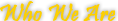ISO 9001:2008 RegisteredHunan Standard Steel Co.,Ltd is as the one of member of Husteel Industry Group, as the professional manufacturer of steel pipe and pipe fittings,which has a complete system of production and sales serving our customers.
Factory          Group

Seamless Steel Pipe

# ASTM A106

 Specifications Range Out Diameter 1/8"- 48" Wall Thickness Schedules 10 through 160, STD, XH and XXH

Standard: ASTM A106M Standard of American Society for Testing&Materials.
Uses: For conveying oil,gas and other fluids.
Chemical Composition(%):

 Standard Grade C Si Mn P S Ni Cr Cu Mo V ASTM A106 A =0.25 =0.10 0.27-0.93 =0.035 =0.035 =0.40 =0.40 =0.40 =0.15 =0.08 B =0.30 =0.10 0.29-1.06 =0.035 =0.035 =0.40 =0.40 =0.40 =0.15 =0.08 C =0.35 =0.10 0.29-1.06 =0.035 =0.035 =0.40 =0.40 =0.40 =0.15 =0.08

Mechanical Properties:

 Standard Grade Tensile strength  (Mpa) Yield strength  (Mpa) ASTM A106 A =330 =205 B =415 =240 C =485 =275